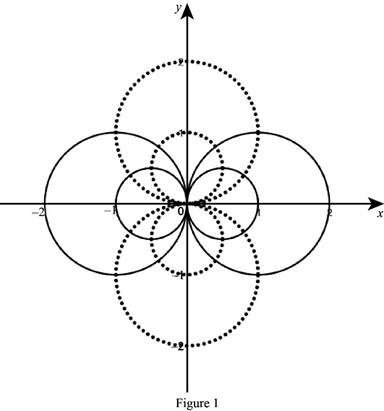# One family of the curves are orthogonal trajectories to the other family.### Single Variable Calculus: Concepts...

4th Edition
James Stewart
Publisher: Cengage Learning
ISBN: 9781337687805### Single Variable Calculus: Concepts...

4th Edition
James Stewart
Publisher: Cengage Learning
ISBN: 9781337687805

#### Solutions

Chapter 3.5, Problem 42E
To determine

## To show: One family of the curves are orthogonal trajectories to the other family.

Expert Solution

### Explanation of Solution

Given:

The equation of the curves x2+y2=ax and x2+y2=by.

Derivative rules:

Chain rule: dydx=dydududx

Proof:

The two family of the circles x2+y2=ax and x2+y2=by. are passing through the point (0,0).

Clearly, the tangent line to the family of curves x2+y2=ax and x2+y2=by at (0,0) is vertical and horizontal line, where a and b are nonzero.

Therefore, the two family of the circles are orthogonal trajectories of each other at (0,0).

Consider two circle is intersect at non zero point (x0,y0).

Substitute (x0,y0) for (x,y) in the family of the circles,

x02+y02=ax0 (1)

x02+y02=by0 (2)

Differentiate x2+y2=ax implicitly with respect to x,

Apply the chain rule and simplify the terms,

2x+[ddy(y2)dydx]=a2x+2ydydx=a2ydydx=a2xdydx=a2x2y

Thus, the derivative of the equation x2+y2=ax is dydx=a2x2y.

That is, the slope of the tangent to x2+y2=ax at (x0,y0) is m1=a2x02y0.

Differentiate x2+y2=by implicitly with respect to x,

ddx(x2+y2)=ddx(by)ddx(x2)+ddx(y2)=bddx(y)2x+ddx(y2)=bdydx

Apply the chain rule and simplify the terms,

2x+[ddy(y2)dydx]=bdydx2x+2ydydx=bdydxdydx(2yb)=2xdydx=2xb2y

Thus, the derivative of the equation x2+y2=ax is dydx=2xb2y.

That is, the slope of the tangent to x2+y2=ax at (x0,y0) is m2=2x0b2y0.

Note: The two tangent lines are orthogonal if the product of their slopes is -1.

m1m2=1(a2x02y0)(2x0b2y0)=1ax02x02=by0+2y02ax0+by0=2(x02+y02)

Substitute the equation (1) and (2),

(x02+y02)+(x02+y02)=2(x02+y02)2(x02+y02)=2(x02+y02)

Therefore, the family of the curves x2+y2=ax and x2+y2=by are orthogonal trajectories to each others.

Hence the required result is proved.

Graph:

The sketch of the family of the circles x2+y2=ax and circles x2+y2=by as shown below in Figure 1.From the graph, it is observed that the two family of the circles x2+y2=ax and x2+y2=by are orthogonal trajectories.

### Have a homework question?

Subscribe to bartleby learn! Ask subject matter experts 30 homework questions each month. Plus, you’ll have access to millions of step-by-step textbook answers!